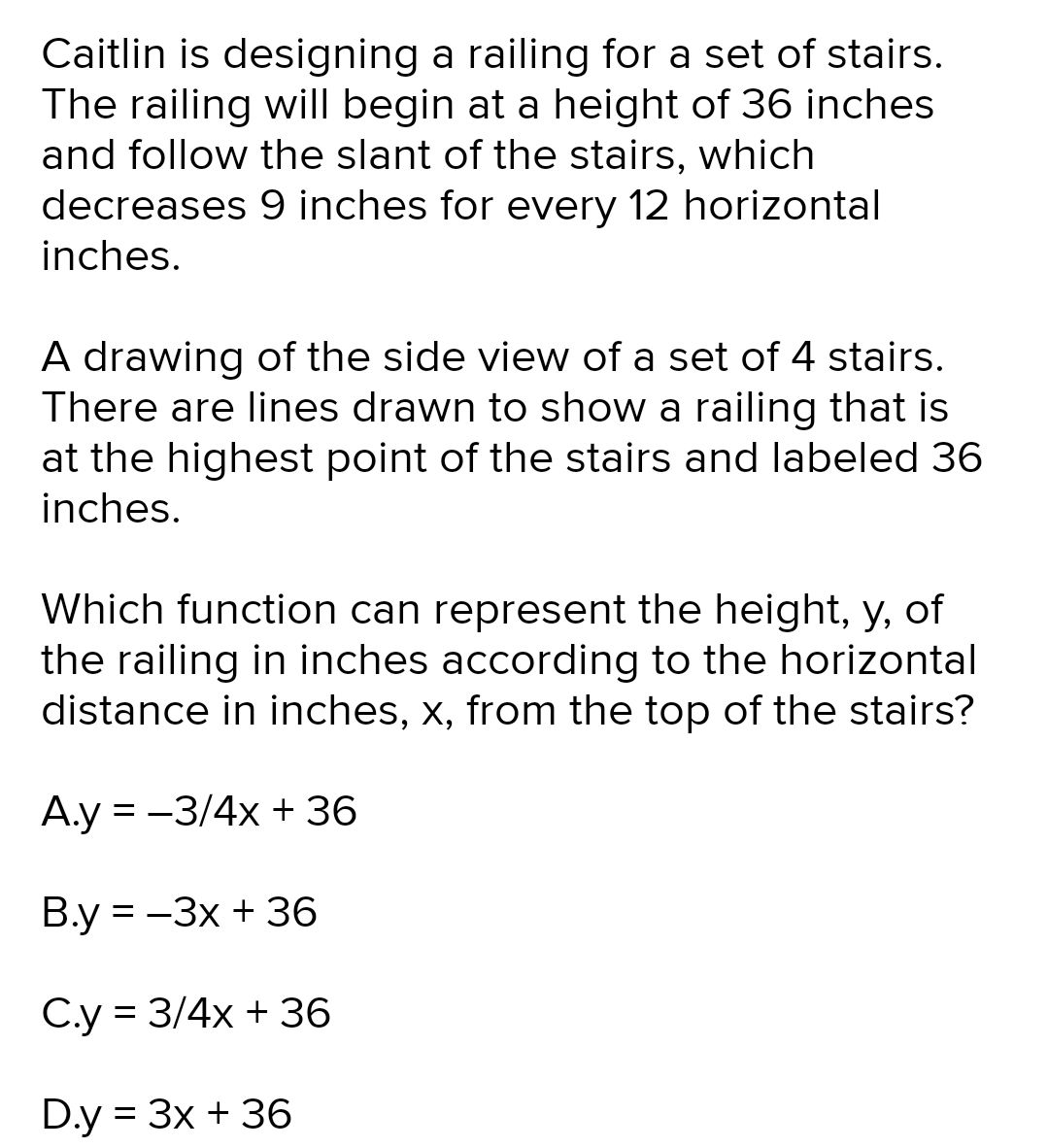### Still have math questions?

Algebra
QuestionCaitlin is designing a railing for a set of stairs. The railing will begin at a height of $$36$$ inches and follow the slant of the stairs, which decreases $$9$$ inches for every $$12$$ horizontal inches.

A drawing of the side view of a set of $$4$$ stairs. There are lines drawn to show a railing that is at the highest point of the stairs and labeled $$36$$ inches.

Which function can represent the height, y, of the railing in inches according to the horizontal distance in inches, $$x ,$$ from the top of the stairs?

A.y $$= - 3 / 4 x + 36$$

B.y $$= - 3 x + 36$$

C.y $$= 3 / 4 x + 36$$

D.y $$= 3 x + 36$$